# How to Count Cells Are Not Blank or Empty in Excel

This post will guide you how to count cells that are not blank or empty in a given range cells using a formula in Excel 2013/2016.How do I count the number of cells that are not blank in a particular range with a VBA macro in Excel. You should know that excel offers several count functions to count the number of cells within a specific range, that meet the defined criteria or conditions in Excel.

If you want to count the number of cells that are not blank or non-empty in a selected range, you can use the COUNTA function or COUNTIF function.

## Method 1: Count Non-Blank or Non-empty Cells with COUNTA Function

Assuming that you have a data list in a range A1:A6, and this range has blank cells as well. And you wish to count cell without blank cells. And you can use a formula based on the COUNTA function like this:

=COUNTA(A1:B6)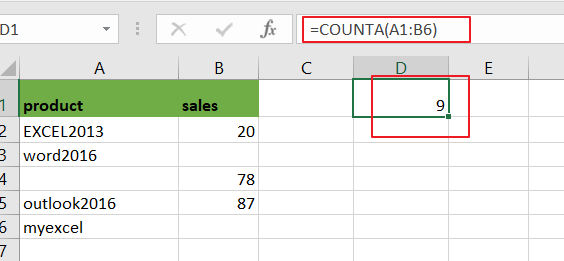The COUNTA function can be used to count the number of cells that contain numbers, text,dates or errors. And it will ignore all empty cells automatically.

## Method 2: Count Non-Blank or Non-empty Cells with COUNTIF Function

You can also use another function called COUNTIF function to achieve the same result of counting the number of cells that are not blank in Excel. For example, using the following formula:

=COUNTIF(A1:B6,”<>”)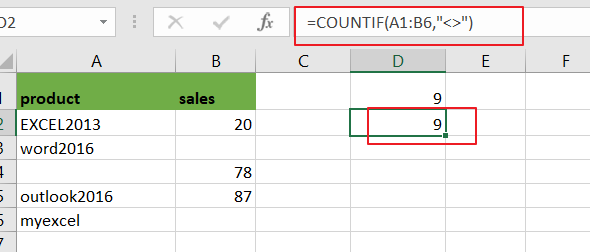From the above image, you would see that using the COUNTIF function to count the number of non-blank or non-empty cells in the range A1:B6 and return the same results as COUNTA function.

## Method 3: Count Non-Blank or Non-empty Cells with VBA Macro

You can also create an newly VBA Macro to count cells that are not empty in a given range in Excel. just do the following steps:

Step1# open your excel workbook and then click on “Visual Basic” command under DEVELOPER Tab, or just press “ALT+F11” shortcut.Step2#  then the “Visual Basic Editor” window will appear.

x
How to Limit Data Entry in a Cell in Excel

Step3# click “Insert” ->”Module” to create a new module.Step4# paste the below VBA code into the code window. Then clicking “Save” button.

```Sub CountCellNotBlank()
Dim ws As Worksheet
Set ws = Worksheets("Sheet7")
ws.Range("D3") = Application.WorksheetFunction.CountA(ws.Range("A1:B6"))
End Sub

```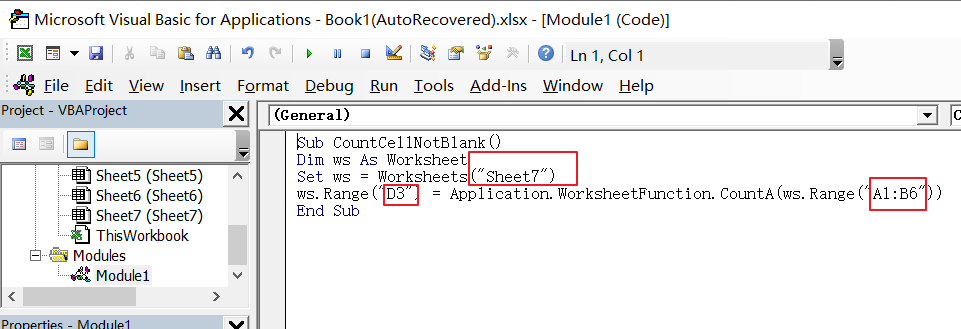Step5: back to the current worksheet, click on `Macros` button under `Code` group. then click `Run` button.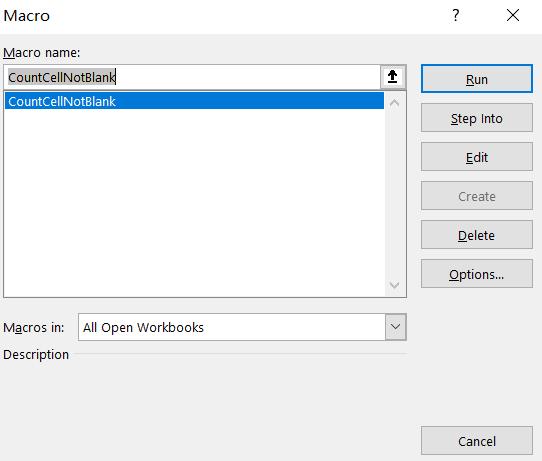Step6: let’s see the result: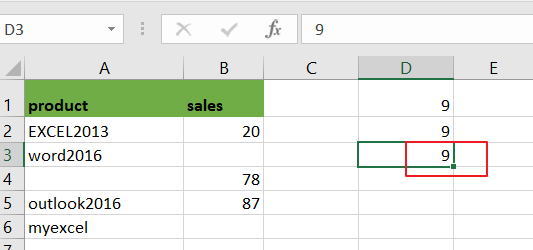### Related Functions

• Excel COUNTIF function
The Excel COUNTIF function will count the number of cells in a range that meet a given criteria. This function can be used to count the different kinds of cells with number, date, text values, blank, non-blanks, or containing specific characters.etc.= COUNTIF (range, criteria)…
• Excel COUNTA function
The Excel COUNTA function counts the number of cells that are not empty in a range. The syntax of the COUNTA function is as below:= COUNTA(value1, [value2],…)…

Related Posts

VBA Macro For VLOOKUP From Another Sheet

In the previous post, you should know that how to fix or remove the #N/A error when using VLOOKUP formula to lookup value from another sheet. And this post will show you how to use VBA code to vlookup data ...

How To Insert Comments in Protected Worksheet in Excel

This post will show you how to allow comments in a protected worksheet in Excel. You can easily to insert comments into cells in a normal worksheet in Excel, but if want to insert a comment in a worksheet that ...

How To Convert Text to Upper Cases(Using VBA) in Excel

This post will show you how to switch from lower case to upper case in Excel. and I am going to show you two different ways of converting text to upper cases using formula or VBA macro in Excel 2013,Excel ...

How To Hide Every Other Row in Excel (Using VBA)

This post will show you how to hide alternate rows or columns in Excel or how to hide every third, fourth, fifth row or column in Excel. If you want to hide every other row in your current worksheet, how ...

How to Disable the Save As Prompt in Excel

This post will show you how to use a VBA Macro to save an Excel file and overwrite any existing file without a prompt so that you are going to get the little window that says file already exists do ...

How to Count Cells that Contain even or odd numbers in Excel

This post will guide you how to count the number of cells that contain odd or even numbers within a range of cells using a formula in Excel 2013/2016.How do I count cells that contain odd numbers through the use ...

How to Count Cells that Contain negative Numbers in Excel

This post will guide you how to count the number of cells that contain negative numbers within a range of cells using a formula in Excel 2013/2016.You can count the number of negative numbers in your data using easy functions ...

How to Count Cells Less Than a Specific Value in Excel

This post will guide you how to count the number of cells less than a particular numeric value in a given range cells using a formula in Excel 2013/2016. How do I count cells that are less than a specific ...

How to Count Cells Greater Than a Specific Value in Excel

This post will guide you how to count the number of cells greater than a particular numeric value in a given range cells using a formula in Excel 2013/2016. How do I count cells that are greater than a specific ...

How to Remove All Extra Spaces and Keep Only One Between Words in Excel

Sometimes when copying something from other type files to excel, there might be two or more spaces display between words. Extra spaces between words are frequently to be seen in excel, and this behavior is very annoying. If we want ...

Sidebar